# Interactive Plots¶

The `cytoflow` package is designed for both scripting and interactive use. As much as I would like the whole world to use data-driven gating and analysis methods, many workflows still require manually specifying gates, and this is most easily done by drawing the gate on a plot.

Fortunately, through the combination of `matplotlib` and the Jupyter notebook, we can have the best of both worlds: interactive REPL and plots that we can point-and-click with.

Clearly, this is best run interactively; but if you’re looking at this notebook online, you should still get a flavor of the package’s capabilities.

First, set up `Jupyter`’s `matplotlib` support, and import the `cytoflow` module. Note the `%matplotlib notebook` instead of `%matplotlib inline`.

```# in some buggy versions of the Jupyter notebook, this needs to be in its OWN CELL.
%matplotlib notebook
```
```import cytoflow as flow

# if your figures are too big or too small, you can scale them by changing matplotlib's DPI
import matplotlib
matplotlib.rc('figure', dpi = 160)
```

Load a few example files, conditioning them on a float variable `Dox`.

```tube1 = flow.Tube(file='data/RFP_Well_A3.fcs', conditions = {"Dox" : 10.0})
tube2 = flow.Tube(file='data/CFP_Well_A4.fcs', conditions = {"Dox" : 1.0})

import_op = flow.ImportOp(conditions = {"Dox" : "float"},
tubes = [tube1, tube2])

ex = import_op.apply()
```

Plot the `Y2-A` channel. We can see there’s a bimodal distribution in one of the tubes.

```flow.HistogramView(channel = "Y2-A",
scale = "logicle",
huefacet = "Dox").plot(ex)
```
```<IPython.core.display.Javascript object>
```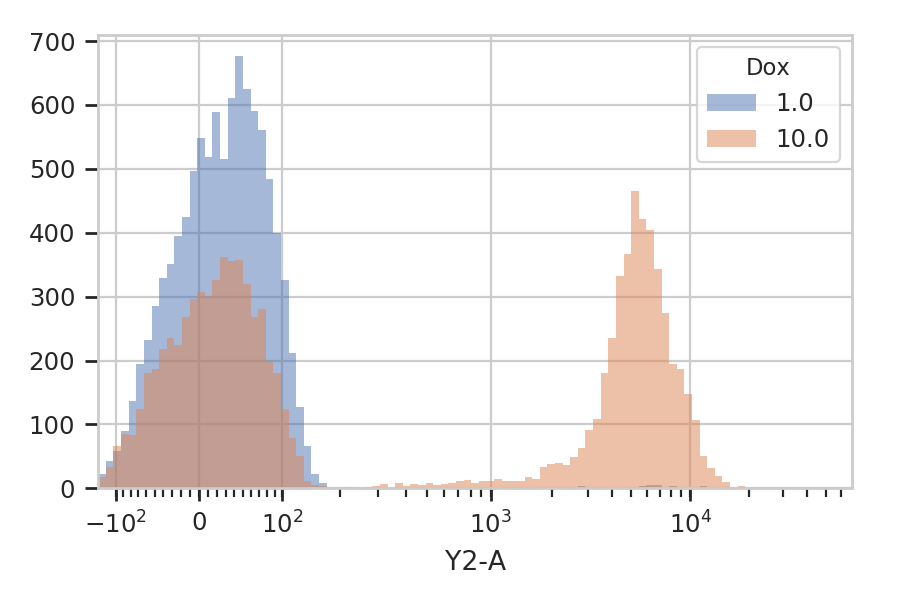Let’s use a `ThresholdOp` to split out the top peak. You can get an interactive plot (the same `HistogramView` as above) by calling `ThresholdOp`’s `default_view()` method. The view that gets returned is linked back to the `ThresholdOp` that produced it: it shows the proper channel, and when you draw a threshold on it the `ThresholdOp` instance’s `threshold` trait gets updated.

One other thing to note: because the `ThresholdOp`’s default view is derived from `HistogramView`, you can use all (well, most!) of the functionality in a regular `HistogramView`. Here, we’ll use the the `huefacet` trait to plot the same multi-colored histogram as above.

As shown below, the steps for using an interactive view are: * Instantiate the operation * Call the operation’s `default_view()` to get the interactive view. * Plot the view. * Set the view’s `interactive` trait to `True`. This step can go before or after calling `plot()`. * Note that the view now shows a cursor (a vertical blue line) that follows your mouse as you move it around the view. Select the threshold you want, then click the mouse button to set it. A fixed blue line appears.

```t = flow.ThresholdOp(name = "Threshold",
channel = "Y2-A")
tv = t.default_view()
tv.huefacet = "Dox"
tv.scale = "logicle"
tv.interactive = True

tv.plot(ex)
```
```<IPython.core.display.Javascript object>
```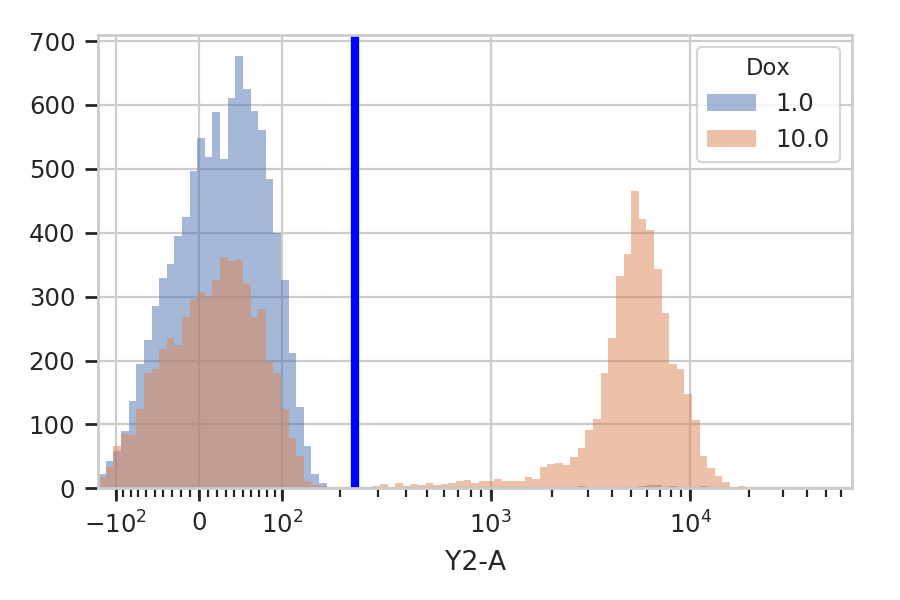After you’ve drawn a threshold on the plot, look at the `ThresholdOp` instance’s `threshold` trait and see that it matches the threshold you drew.

```t.threshold
```
```235.97817044140453
```

You can then apply the newly parameterized operation to the data set.

```ex2 = t.apply(ex)
flow.HistogramView(channel = "Y2-A",
scale = "logicle",
huefacet = "Dox",
yfacet = "Threshold").plot(ex2)
```
```<IPython.core.display.Javascript object>
```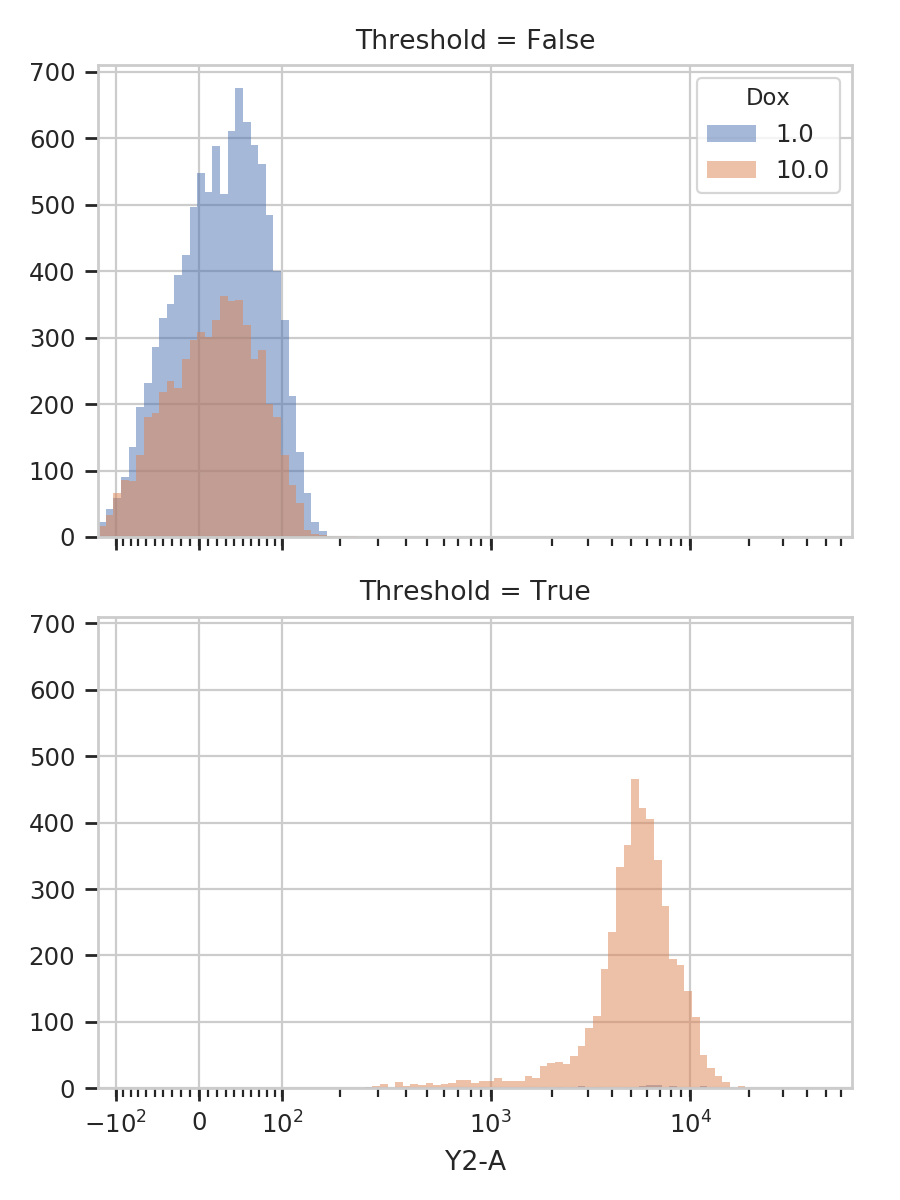We can use a similar strategy with the `RangeOp`. The setup is very much the same; but instead of a single click, drag the cursor to set the range.

Also note that we can compress the invocation by passing the parameters to `default_view()` (as we would to a constructor.)

```r = flow.RangeOp(name = "Range",
channel = "Y2-A")

r.default_view(huefacet = "Dox",
scale = "logicle",
interactive = True).plot(ex)
```
```<IPython.core.display.Javascript object>
```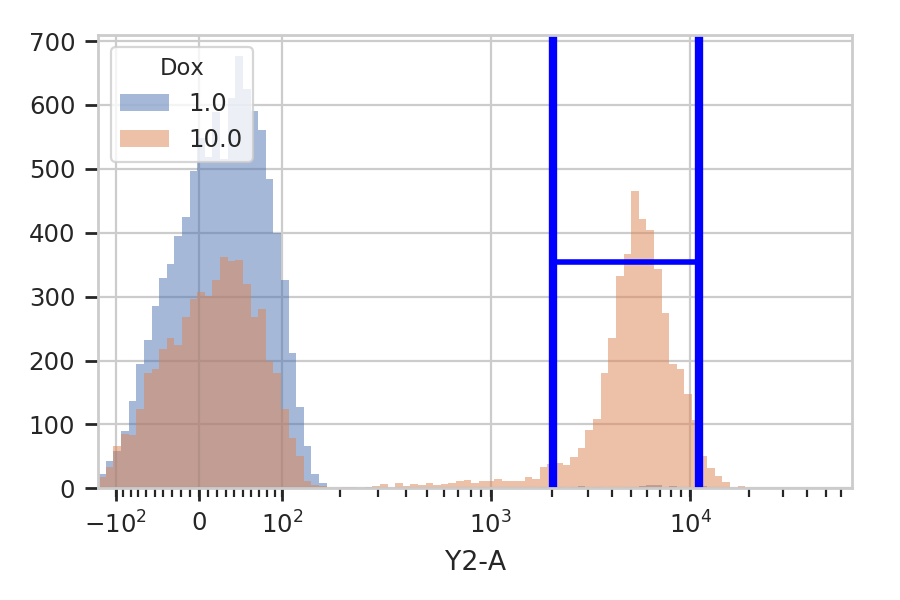```r.low, r.high
```
```(2007.3540593264756, 11086.840684744158)
```

You can also draw ranges on 2D plots. Again, drag the cursor to draw a range.

```r2d = flow.Range2DOp(name = "Range2D",
xchannel = "V2-A",
ychannel = "Y2-A")

r2d.default_view(huefacet = "Dox",
xscale = "logicle",
yscale = "logicle",
interactive = True).plot(ex)
```
```<IPython.core.display.Javascript object>
```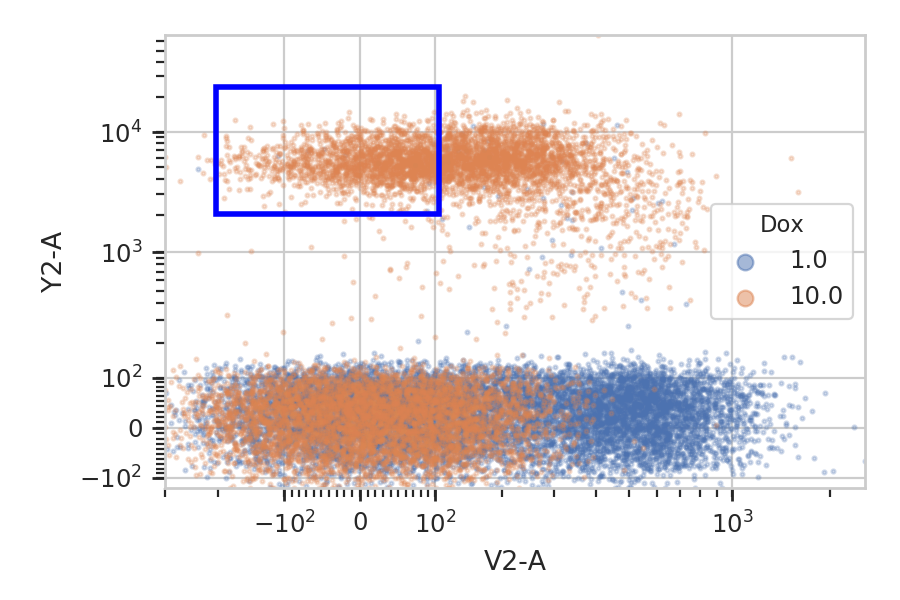```r2d.xlow, r2d.xhigh, r2d.ylow, r2d.yhigh
```
```(-203.01634412009793,
104.94315196137035,
2038.5106539405642,
24562.81382553335)
```

You can specify a polygon this way too. Unforunately, the JavaScript link between the Jupyter notebook and the Python kernel is a little slow, so the performance here is … not ideal. Be patient.

Single click to set vertices; click the first vertex a second time to close the polygon.

```p = flow.PolygonOp(name = "Polygon",
xchannel = "V2-A",
ychannel = "Y2-A")

pv = p.default_view(huefacet = "Dox",
xscale = "logicle",
yscale = "logicle",
interactive = True)

pv.plot(ex)
```
```<IPython.core.display.Javascript object>
```